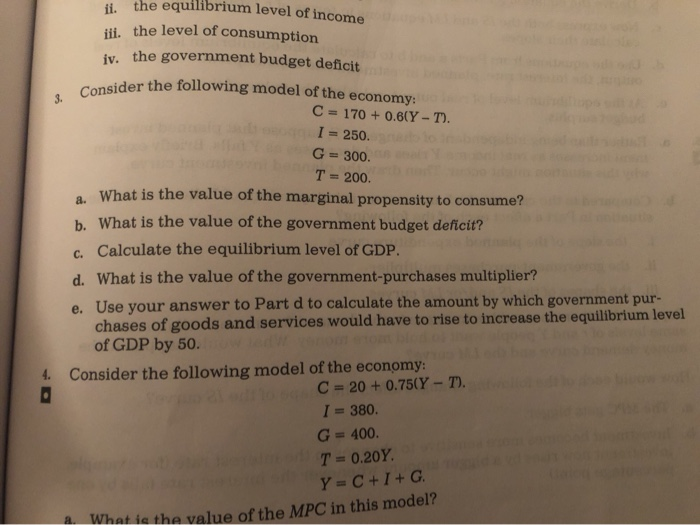# Question 3. Consider hi the equilibrium level of income iii. the level of consumption iv. the government budget deficit Consider the following model of the economy: C = 170 + 0.60Y-T). I = 250. G = 300. T = 200. a. What is the value of the marginal propensity to consume? b. What is the value of the government budget deficit? c. Calculate the equilibrium level of GDP. 3. Consider hi the equilibrium level of income iii. the level of consumption iv. the government budget deficit Consider the following model of the economy: C = 170 + 0.60Y-T). I = 250. G = 300. T = 200. a. What is the value of the marginal propensity to consume? b. What is the value of the government budget deficit? c. Calculate the equilibrium level of GDP. d. What is the value of the government-purchases multiplier? e. Use your answer to Part d to calculate the amount by which government pur chases of goods and services would have to rise to increase the equilibrium level of GDP by 50. 4. Consider the following model of the economy: C = 20 +0.750Y-T). I = 380. G = 400. T = 0.20Y. y=C+I+ G. What is the value of the MPC in this model?